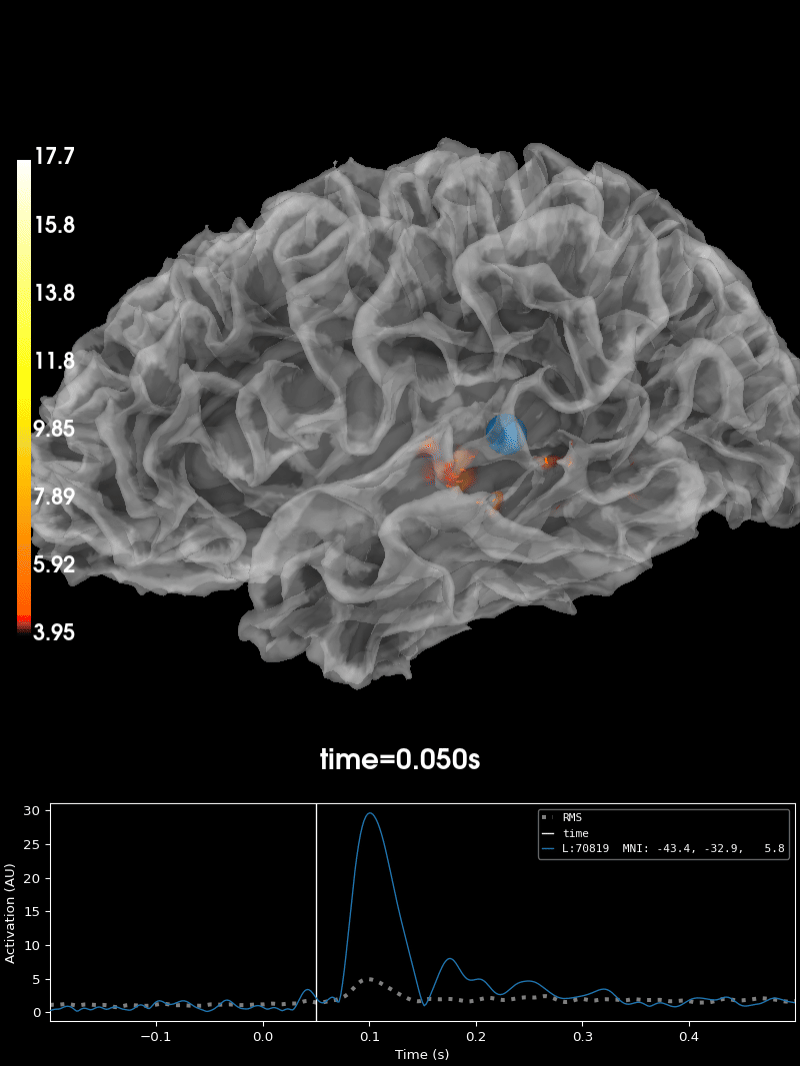# Plotting the full vector-valued MNE solution#

The source space that is used for the inverse computation defines a set of dipoles, distributed across the cortex. When visualizing a source estimate, it is sometimes useful to show the dipole directions in addition to their estimated magnitude. This can be accomplished by computing a `mne.VectorSourceEstimate` and plotting it with `stc.plot`, which uses `plot_vector_source_estimates()` under the hood rather than `plot_source_estimates()`.

It can also be instructive to visualize the actual dipole/activation locations in 3D space in a glass brain, as opposed to activations imposed on an inflated surface (as typically done in `mne.SourceEstimate.plot()`), as it allows you to get a better sense of the underlying source geometry.

```# Author: Marijn van Vliet <w.m.vanvliet@gmail.com>
#
```
```import numpy as np
import mne
from mne.datasets import sample

print(__doc__)

data_path = sample.data_path()
subjects_dir = data_path / "subjects"
smoothing_steps = 7

meg_path = data_path / "MEG" / "sample"
fname_evoked = meg_path / "sample_audvis-ave.fif"
evoked = mne.read_evokeds(fname_evoked, condition=0, baseline=(None, 0))

fname_inv = meg_path / "sample_audvis-meg-oct-6-meg-inv.fif"

# Apply inverse solution, set pick_ori='vector' to obtain a
# :class:`mne.VectorSourceEstimate` object
snr = 3.0
lambda2 = 1.0 / snr**2
stc = apply_inverse(evoked, inv, lambda2, "dSPM", pick_ori="vector")

# Use peak getter to move visualization to the time point of the peak magnitude
_, peak_time = stc.magnitude().get_peak(hemi="lh")
```
```Reading /home/circleci/mne_data/MNE-sample-data/MEG/sample/sample_audvis-ave.fif ...
Read a total of 4 projection items:
PCA-v1 (1 x 102) active
PCA-v2 (1 x 102) active
PCA-v3 (1 x 102) active
Average EEG reference (1 x 60) active
Found the data of interest:
t =    -199.80 ...     499.49 ms (Left Auditory)
0 CTF compensation matrices available
nave = 55 - aspect type = 100
Projections have already been applied. Setting proj attribute to True.
Applying baseline correction (mode: mean)
Reading inverse operator decomposition from /home/circleci/mne_data/MNE-sample-data/MEG/sample/sample_audvis-meg-oct-6-meg-inv.fif...
[done]
[done]
305 x 305 full covariance (kind = 1) found.
Read a total of 4 projection items:
PCA-v1 (1 x 102) active
PCA-v2 (1 x 102) active
PCA-v3 (1 x 102) active
Average EEG reference (1 x 60) active
22494 x 22494 diagonal covariance (kind = 2) found.
22494 x 22494 diagonal covariance (kind = 6) found.
22494 x 22494 diagonal covariance (kind = 5) found.
Did not find the desired covariance matrix (kind = 3)
Computing patch statistics...
[done]
Computing patch statistics...
[done]
Read a total of 4 projection items:
PCA-v1 (1 x 102) active
PCA-v2 (1 x 102) active
PCA-v3 (1 x 102) active
Average EEG reference (1 x 60) active
Source spaces transformed to the inverse solution coordinate frame
Preparing the inverse operator for use...
Scaled noise and source covariance from nave = 1 to nave = 55
Created the regularized inverter
Created an SSP operator (subspace dimension = 3)
Created the whitener using a noise covariance matrix with rank 302 (3 small eigenvalues omitted)
Computing noise-normalization factors (dSPM)...
[done]
Applying inverse operator to "Left Auditory"...
Picked 305 channels from the data
Computing inverse...
Eigenleads need to be weighted ...
Computing residual...
Explained  59.4% variance
dSPM...
[done]
```

Plot the source estimate:

```brain = stc.plot(
initial_time=peak_time,
hemi="lh",
subjects_dir=subjects_dir,
smoothing_steps=smoothing_steps,
)

# You can save a brain movie with:
# brain.save_movie(time_dilation=20, tmin=0.05, tmax=0.16, framerate=10,
#                  interpolation='linear', time_viewer=True)
```